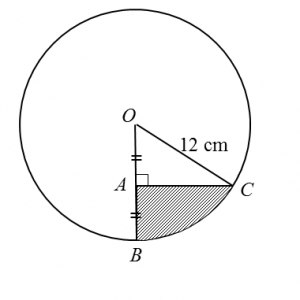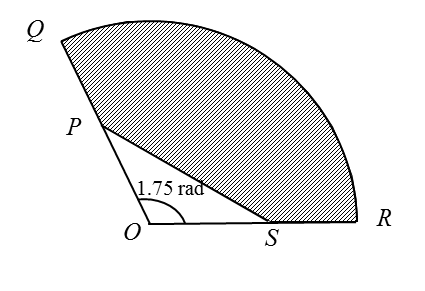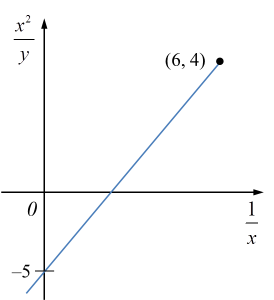# Short Questions (Question 7 & 8)

Question 7:
Diagram below shows a circle with centre O and radius 12 cm.Given that A, B and C are points such that OA = AB and  ∠OAC = 90°, find
(a)   ∠BOC, in radians,
(b)  the area, in cm2, of the shaded region.

Solution:
(a) For triangle OAC,
cos  ∠AOC = 6/12
ÐAOC = 1.047 rad (change calculator to Rad mode)
ÐBOC = 1.047 rad

(b)
Area of the shaded region
= Area of BOC – Area of triangle AOC
½ (12)2 (1.047) – ½ (6) (12) sin 1.047 (change calculator to Rad mode)
= 75.38 – 31.17
= 44.21 cm2

Question 8:
Diagram below shows a sector QOR of a circle with centre O.It is given that PS = 8 cm and QP = PO= OS = SR = 5 cm.
Find
(a) the length, in cm, of the arc QR,
(b) the area, in cm2, of the shaded region.

Solution:
(a) Length of arc QR = θ = 10 (1.75) = 17.5 cm

(b)
Area of the shaded region
= Area of sector QOR – Area of triangle POS
½ (10)2 (1.75) – ½ (5) (5) sin 1.75 (change calculator to Rad mode)
= 87.5 – 12.30
= 75.2 cm2

# 9.7 Second-Order Differentiation, Turning Points, Maximum and Minimum Points (Examples)

Example 1 (Maximum Value of Quadratic Function)
Given that y = 3x (4 – x), calculate
(a) the value of x when y is a maximum,
(b) the maximum value of y.

Solution:
(a)

(b)

Example 2 (Determine the Turning Points and Second Derivative Test)
Find the coordinates of the turning points on the curve y = 2x3 + 3x2 – 12x + 7 and determine the nature of these turning points.

Solution:

6x2 + 6x – 12 = 0
x2 + x – 2 = 0
(x – 1) (x + 2) = 0
x = 1 or x = –2

When x = 1
y = 2(1)3 + 3(1)2 – 12(11) + 7
y = 0
(1, 0) is a turning point.

When x = –2
y = 2(–2)3 + 3(–2)2 – 12(–2) + 7
y = 27
(–2, 27) is a turning point.

Hence, the turning point (1, 0) is a minimum point.

Hence, the turning point (–2, 27) is a maximum point.

# SPM Practice Question 2

Question 2:
The third term and the sixth term of a geometric progression are 24 and $7\frac{1}{9}$ respectively. Find
(a) the first term and the common ratio,
(b) the sum of the first five terms,
(c) the sum of the first n terms with n is very big approaching rn ≈ 0.

Solution:
(a)

(b)

(c)

Therefore, sum of the first n terms with n is very big approaching rn ≈ 0 is 162.

# Short Questions (Question 7 & 8)

Question 7:
The masses of mangoes in a stall have a normal distribution with a mean of 200 g and a standard deviation of 30 g.
(a) Find the mass, in g, of a mango whose z-score is 0.5.
(b) If a mango is chosen at random, find the probability that the mango has a mass of at least 194 g.

Solution:
µ = 200 g
σ = 30 g
Let X be the mass of a mango.

(a)
$\begin{array}{l}\frac{X-200}{30}=0.5\\ X=0.5\left(30\right)+200\\ X=215g\end{array}$

(b)
$\begin{array}{l}P\left(X\ge 194\right)\\ =P\left(Z\ge \frac{194-200}{30}\right)\\ =P\left(Z\ge -0.2\right)\\ =1-P\left(Z>0.2\right)\\ =1-0.4207\\ =0.5793\end{array}$

Question 8:
Diagram below shows a standard normal distribution graph.The probability represented by the area of the shaded region is 0.3238.
(a) Find the value of k.
(b) X is a continuous random variable which is normally distributed with a mean of 80 and variance of 9.
Find the value of X when the z-score is k.

Solution:
(a)
P(Z > k) = 0.5 – 0.3238
= 0.1762
k = 0.93

(b)
µ = 80,
σ2 = 9, σ = 3
$\begin{array}{l}\frac{X-80}{3}=0.93\\ X=3\left(0.93\right)+80\\ X=82.79\end{array}$

# Short Questions (Question 5 & 6)

Question 5:
Diagram below shows the graph of a binomial distribution of X.

(a) the value of h,
(b) P (X ≥ 3)

Solution:
(a)
P (X = 0) + P (X = 1) + P (X = 2) + P (X = 3) + P (X = 4) = 1
$\begin{array}{l}\frac{1}{16}+\frac{1}{4}+h+\frac{1}{4}+\frac{1}{16}=1\\ h=1-\frac{5}{8}\\ h=\frac{3}{8}\end{array}$

(b)
P (X ≥ 3) = P (X = 3) + P (X = 4)
$P\left(X\ge 3\right)=\frac{1}{4}+\frac{1}{16}=\frac{5}{16}$

Question 6:
The random variable X represents a binomial distribution with 10 trails and the probability of success is ¼.
(a) the standard deviation of the distribution,
(b) the probability that at least one trial is success.

Solution:
(a)
n = 10, p = ¼
$\begin{array}{l}\text{Standard deviation}=\sqrt{npq}\\ \text{}=\sqrt{10×\frac{1}{4}×\frac{3}{4}}\\ \text{}=1.875\end{array}$

(b)
$\begin{array}{l}P\left(X=r\right)=C{}_{r}{\left(\frac{1}{4}\right)}^{r}{\left(\frac{3}{4}\right)}^{10-r}\\ P\left(X\ge 1\right)\\ =1-P\left(X<1\right)\\ =1-P\left(X=0\right)\\ =1-C{}_{0}{\left(\frac{1}{4}\right)}^{0}{\left(\frac{3}{4}\right)}^{10}\\ =0.9437\end{array}$

# SPM Practice 2 (Question 11 & 12)

Question 11 (3 marks):
Diagram 6 shows the graph of a straight lineDiagram 11

Based on Diagram 6, express y in terms of x.

Solution:

Question 12 (3 marks):
The variables x and y are related by the equation $y=x+\frac{r}{{x}^{2}}$ , where r is a constant. Diagram 8 shows a straight line graph obtained by plottingDiagram 12

Express h in terms of p and r.

Solution:

# SPM Practice 2 (Linear Law) – Question 1

Question 1 (10 marks):
Use a graph to answer this question.
Table 1 shows the values of two variables, x and y, obtained from an experiment. A straight line will be obtained when a graph of is plotted.(a) Based on Table 1, construct a table for the values of

(c) Using the graph in 1(b)
(i) find the value of y when x = 2.7,
(ii) express y in terms of x.

Solution:
(a)(b)(c)(i)

(c)(ii)

# SPM Practice 3 (Linear Law) – Question 6

Question 6
The table below shows the values of two variables, x and y, obtained from an experiment. The variables x and y are related by the equation $\frac{a}{y}=\frac{b}{x}+1$ , where k and p are constants.

(a) Based on the table above, construct a table for the values of $\frac{1}{x}$ and $\frac{1}{y}$ . Plot $\frac{1}{y}$ against $\frac{1}{x}$ , using a scale of  2 cm to 0.1 unit on the $\frac{1}{x}$ - axis and  2 cm to 0.2 unit on the $\frac{1}{y}$ - axis. Hence, draw the line of best fit.
(b) Use the graph from  (b)  to find the value of
(i)  a,
(ii)  b.

Solution

Step 1 : Construct a table consisting X and Y.

Step 2 : Plot a graph of Y against X, using the scale given and draw a line of best fit

Steps to draw line of best fit - Click here

Step 3 : Calculate the gradient, m, and the Y-intercept, c, from the graph

Step 4 : Rewrite the original equation given and reduce it to linear form

Step 5 : Compare with the values of m and c obtained, find the values of the unknown required

# SPM Practice 3 (Linear Law) – Question 5

Question 5
The following table shows the corresponding values of two variables, x and y, that are related by the equation $y=p{k}^{\sqrt{x}}$ , where p and k are constants.

(a) Plot $log 10 y$ against $x$  .  Hence, draw the line of best fit

(b) Use your graph in (a) to find the values of p and k.

# SPM Practice 2 (Linear Law) – Question 4

Question 4
The table below shows the corresponding values of two variables, x and y, that are related by the equation $y=qx+\frac{p}{qx}$ , where p and q are constants.

One of the values of y is incorrectly recorded.
(a) Using scale of 2 cm to 5 units on the both axis, plot the graph of xy against ${x}^{2}$  .  Hence, draw the line of best fit

(b) Use your graph in (a) to answer the following questions:
(i) State the values of y which is incorrectly recorded and determine its actual value.
(ii) Find the value of p and of q.

Solution
Step 1 : Construct a table consisting X and Y.

Step 2 : Plot a graph of Y against X, using the scale given and draw a line of best fit

Steps to draw line of best fit - Click here

(b) (i) State the values of y which is incorrectly recorded and determine its actual value.

Step 3 : Calculate the gradient, m, and the Y-intercept, c, from the graph

Step 4 : Rewrite the original equation given and reduce it to linear form

Step 5 : Compare with the values of m and c obtained, find the values of the unknown required